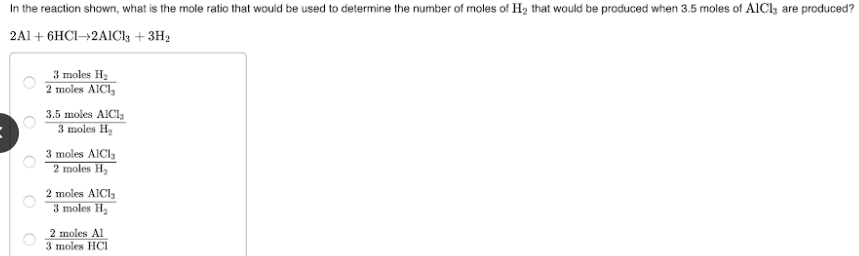# In the reaction shown, what is the mole ratio that would be used to determine the number of moles of H2 that would be produced when 3.5 moles of AlCl3 are produced? 2 Al + 6 HCl → 2 AlCl3 + 3 H2 a. 3 moles H2 / 2 moles AlCl3 b. 3.5 moles AlCl3 / 3 moles H2 c. 3 moles AlCl3 / 2 moles H2 d. 2 moles AlCl3 / 3 moles H2 e. 2 moles Al / 3 moles HCl